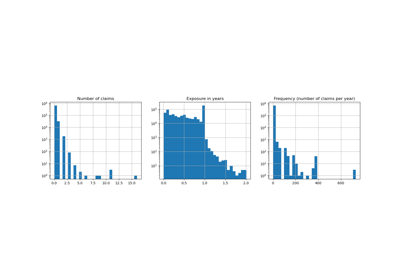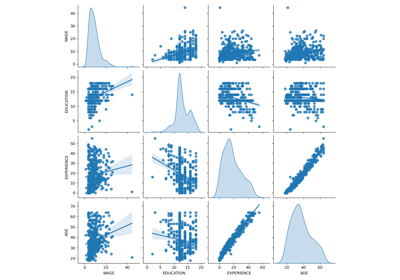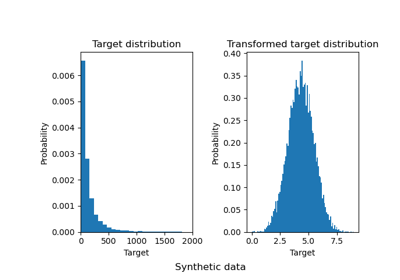# sklearn.compose.TransformedTargetRegressor¶

class sklearn.compose.TransformedTargetRegressor(regressor=None, *, transformer=None, func=None, inverse_func=None, check_inverse=True)[source]

Meta-estimator to regress on a transformed target.

Useful for applying a non-linear transformation to the target y in regression problems. This transformation can be given as a Transformer such as the QuantileTransformer or as a function and its inverse such as np.log and np.exp.

The computation during fit is:

regressor.fit(X, func(y))


or:

regressor.fit(X, transformer.transform(y))


The computation during predict is:

inverse_func(regressor.predict(X))


or:

transformer.inverse_transform(regressor.predict(X))


Read more in the User Guide.

New in version 0.20.

Parameters:
regressorobject, default=None

Regressor object such as derived from RegressorMixin. This regressor will automatically be cloned each time prior to fitting. If regressor is None, LinearRegression is created and used.

transformerobject, default=None

Estimator object such as derived from TransformerMixin. Cannot be set at the same time as func and inverse_func. If transformer is None as well as func and inverse_func, the transformer will be an identity transformer. Note that the transformer will be cloned during fitting. Also, the transformer is restricting y to be a numpy array.

funcfunction, default=None

Function to apply to y before passing to fit. Cannot be set at the same time as transformer. The function needs to return a 2-dimensional array. If func is None, the function used will be the identity function.

inverse_funcfunction, default=None

Function to apply to the prediction of the regressor. Cannot be set at the same time as transformer. The function needs to return a 2-dimensional array. The inverse function is used to return predictions to the same space of the original training labels.

check_inversebool, default=True

Whether to check that transform followed by inverse_transform or func followed by inverse_func leads to the original targets.

Attributes:
regressor_object

Fitted regressor.

transformer_object

Transformer used in fit and predict.

n_features_in_int

Number of features seen during fit.

feature_names_in_ndarray of shape (n_features_in_,)

Names of features seen during fit. Defined only when X has feature names that are all strings.

New in version 1.0.

sklearn.preprocessing.FunctionTransformer

Construct a transformer from an arbitrary callable.

Notes

Internally, the target y is always converted into a 2-dimensional array to be used by scikit-learn transformers. At the time of prediction, the output will be reshaped to a have the same number of dimensions as y.

Examples

>>> import numpy as np
>>> from sklearn.linear_model import LinearRegression
>>> from sklearn.compose import TransformedTargetRegressor
>>> tt = TransformedTargetRegressor(regressor=LinearRegression(),
...                                 func=np.log, inverse_func=np.exp)
>>> X = np.arange(4).reshape(-1, 1)
>>> y = np.exp(2 * X).ravel()
>>> tt.fit(X, y)
TransformedTargetRegressor(...)
>>> tt.score(X, y)
1.0
>>> tt.regressor_.coef_
array([2.])


Methods

 fit(X, y, **fit_params) Fit the model according to the given training data. get_params([deep]) Get parameters for this estimator. predict(X, **predict_params) Predict using the base regressor, applying inverse. score(X, y[, sample_weight]) Return the coefficient of determination of the prediction. set_params(**params) Set the parameters of this estimator.
fit(X, y, **fit_params)[source]

Fit the model according to the given training data.

Parameters:
X{array-like, sparse matrix} of shape (n_samples, n_features)

Training vector, where n_samples is the number of samples and n_features is the number of features.

yarray-like of shape (n_samples,)

Target values.

**fit_paramsdict

Parameters passed to the fit method of the underlying regressor.

Returns:
selfobject

Fitted estimator.

get_params(deep=True)[source]

Get parameters for this estimator.

Parameters:
deepbool, default=True

If True, will return the parameters for this estimator and contained subobjects that are estimators.

Returns:
paramsdict

Parameter names mapped to their values.

property n_features_in_

Number of features seen during fit.

predict(X, **predict_params)[source]

Predict using the base regressor, applying inverse.

The regressor is used to predict and the inverse_func or inverse_transform is applied before returning the prediction.

Parameters:
X{array-like, sparse matrix} of shape (n_samples, n_features)

Samples.

**predict_paramsdict of str -> object

Parameters passed to the predict method of the underlying regressor.

Returns:
y_hatndarray of shape (n_samples,)

Predicted values.

score(X, y, sample_weight=None)[source]

Return the coefficient of determination of the prediction.

The coefficient of determination $$R^2$$ is defined as $$(1 - \frac{u}{v})$$, where $$u$$ is the residual sum of squares ((y_true - y_pred)** 2).sum() and $$v$$ is the total sum of squares ((y_true - y_true.mean()) ** 2).sum(). The best possible score is 1.0 and it can be negative (because the model can be arbitrarily worse). A constant model that always predicts the expected value of y, disregarding the input features, would get a $$R^2$$ score of 0.0.

Parameters:
Xarray-like of shape (n_samples, n_features)

Test samples. For some estimators this may be a precomputed kernel matrix or a list of generic objects instead with shape (n_samples, n_samples_fitted), where n_samples_fitted is the number of samples used in the fitting for the estimator.

yarray-like of shape (n_samples,) or (n_samples, n_outputs)

True values for X.

sample_weightarray-like of shape (n_samples,), default=None

Sample weights.

Returns:
scorefloat

$$R^2$$ of self.predict(X) wrt. y.

Notes

The $$R^2$$ score used when calling score on a regressor uses multioutput='uniform_average' from version 0.23 to keep consistent with default value of r2_score. This influences the score method of all the multioutput regressors (except for MultiOutputRegressor).

set_params(**params)[source]

Set the parameters of this estimator.

The method works on simple estimators as well as on nested objects (such as Pipeline). The latter have parameters of the form <component>__<parameter> so that it’s possible to update each component of a nested object.

Parameters:
**paramsdict

Estimator parameters.

Returns:
selfestimator instance

Estimator instance.

## Examples using sklearn.compose.TransformedTargetRegressor¶Poisson regression and non-normal loss

Poisson regression and non-normal lossCommon pitfalls in the interpretation of coefficients of linear models

Common pitfalls in the interpretation of coefficients of linear modelsEffect of transforming the targets in regression model

Effect of transforming the targets in regression model